International
Tables for
Crystallography
Volume D
Physical properties of crystals
Edited by A. Authier

International Tables for Crystallography (2006). Vol. D, ch. 1.4, pp. 101-102

Section 1.4.3.1. General remarks

H. Küppersa*

aInstitut für Geowissenshaften, Universität Kiel, Olshausenstrasse 40, D-24098 Kiel, Germany
Correspondence e-mail: kueppers@min.uni-kiel.de

1.4.3.1. General remarks

| top | pdf |

Although the strain tensorand the thermal expansion tensorin general contain components with(shear strains), in practice only longitudinal effects, i.e. relative length changes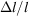with temperature changes ΔT, are measured along different directions and the results are later transformed to a common coordinate system. Diffraction methods directly yield this ratio. Other measuring techniques require separate measurements of Δl and l. The error in the measurement of l can usually be neglected. Thus, the accuracies of Δl and ΔT limit the accuracy of thermal expansion coefficients. The temperature interval ΔT is determined by two measurements of temperatures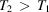, with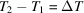. To increase the accuracy of the difference ΔT, this interval should be large. The measured thermal expansion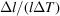is usually assigned to a temperature at the midpoint of the temperature interval,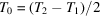. This procedure is only justified if thermal expansion does not depend on temperature.

Since, in fact, thermal expansion depends on temperature, in principle, smaller intervals should be chosen, which, in turn, enlarge the error of ΔT. Here, a compromise has to be made. Sometimes, after completion of a first run and after reviewing the preliminary course of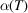, it is necessary to repeat some measurements using smaller temperature intervals in temperature ranges with large curvatures.

The more-or-less curved course of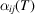is usually fitted by polynomials in powers of temperature. Here, those T terms should be selected that are physically meaningful in the particular temperature range. For the low-temperature behaviour of a metal, a polynomial of type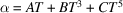should be chosen. For minerals at higher temperatures, a polynomial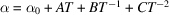is used (Saxena & Shen, 1992).

Temperature is usually measured by thermocouples and, in the cases of optical or electrical measurements (Sections 1.4.3.3and 1.4.3.4) and at low temperatures also by platinum resistance thermometers. Above 1100 K, optical pyrometers can be used.

In order to measure the thermal expansion of a crystal, at least as many independent measurements are necessary as the tensor has independent components (fourth column in Table 1.4.1.1). It is advisable, however, to carry out more measurements than are necessary. In this case (of redundancy), a best' set of tensor components is to be determined by least-squares methods as described below.

Let us assume the most general case of a triclinic crystal, where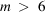independent measurements of thermal expansions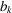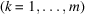were performed along m different directions with direction cosines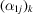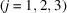with respect to the chosen coordinate system. Each measurementis related to the six unknown tensor components(to be determined) by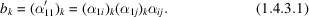If theare replaced by, using Voigt's one-index notation (Section 1.1.4.10.2), thenrepresents an overdetermined inhomogeneous system of m linear equations for the six unknowns. The coefficients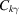, forming an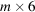matrix, are products containing direction cosines according to (1.4.3.1). The solution is obtained after several matrix calculations which are indicated by the formula (Nye, 1985)where a superscript t' means transposed.

Instead of determining the tensor components of a triclinic or monoclinic crystal in a direct way, as outlined above, it is also possible to determine first the temperature change of the crystallographic unit cell and then, by formulae given e.g. by Schlenker et al. (1978), to deduce the tensor components. The direct approach is recommended, however, for reasons of the propagation of errors (Jessen & Küppers, 1991).

The experimental techniques of measuring relative length changesthat are most widely used include diffraction, optical interferometry, pushrod dilatometry and electrical capacitance methods. If the specimens available are very small and/or irregular in shape, only diffraction methods can be used. The other methods require single-crystal parallelepipedal samples with at least 5 mm side lengths.

References

Jessen, S. M. & Küppers, H. (1991). The precision of thermal-expansion tensors of triclinic and monoclinic crystals. J. Appl. Cryst. 24, 239–242.Google Scholar
Nye, J. F. (1985). Physical properties of crystals. Oxford: Clarendon Press.Google Scholar
Saxena, S. K. & Shen, G. (1992). Assessed data on heat capacity, thermal expansion, and compressibility of some oxides and silicates. J. Geophys. Res. 97, 19813–19825.Google Scholar
Schlenker, J. L., Gibbs, G. V. & Boisen, M. B. (1978). Strain-tensor components expressed in terms of lattice parameters. Acta Cryst. A34, 52–54.Google Scholar